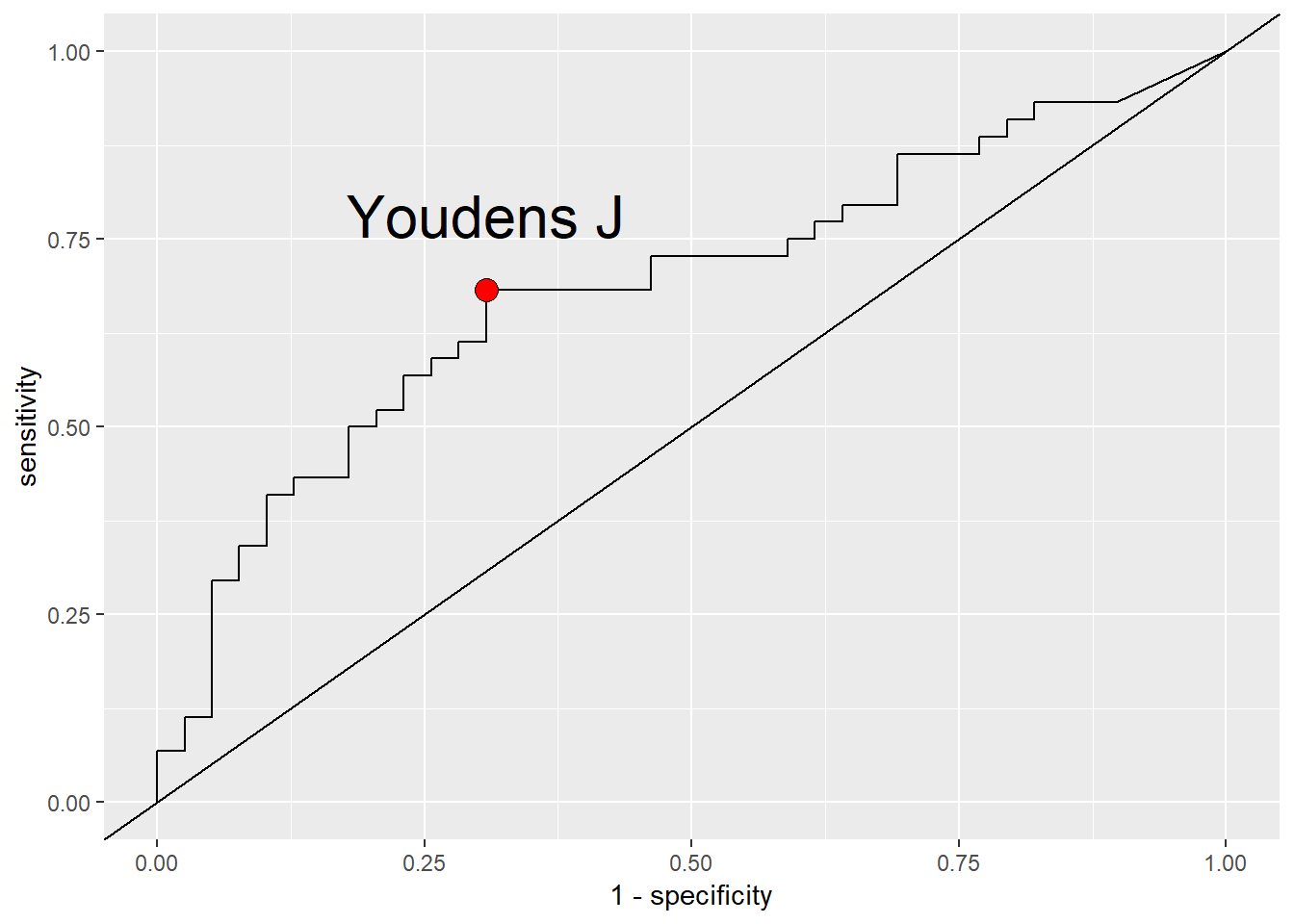# Summary measures of predictive power associated with logistic regression models of disease risk### Abstract

For an ordinary least squares regression model, the coefficient of determination R2 describes the proportion (or percentage) of variance of the response variable explained by the model, and is a widely-accepted summary measure of predictive power. A number of R2-analogues are available as summary measures of predictive power for logistic regression models, including models of disease risk. Tjur’s R2 and McFadden’s R2 are of particular interest in this context. Both these R2-analogues have transparent derivations, which reveal that they apply to different aspects of model evaluation: Tjur’s R2 is a measure of separation between (known) actual states (e.g., gold standard determinations of “healthy” or “diseased” status) whereas McFadden’s R2 is a measure of separation between predicted states (e.g., forecasts of disease status based on models of disease risk). This clarifies their interpretation in the context of evaluation of logistic regression models of disease risk. In addition, versions of both Tjur’s R2 and McFadden’s R2 may be obtained from analyses of disease risk that are not preceded by logistic regression analysis.Tjur’s R2 and McFadden’s R2 are shown to be useful, distinct summary measures of predictive power for epidemiological models of disease risk.

Publication
Phytopathology 109: In Press
Date